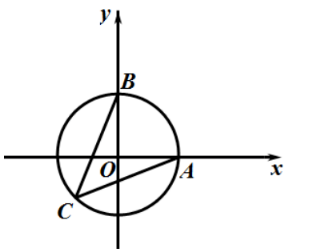【答案】 $1-\sqrt{2} \# \#-\sqrt{2}+1$

【解析】 因为 $|A B|=\sqrt{2}$, 又 $|O A|=|O B|=1$, 所以 $|O A|^2+|O B|^2=|A B|^2$, 所以 $\angle A O B=\frac{\pi}{2}$ 以 $O$ 为原点, $O A, O B$ 所在直线为 $x, y$ 轴建立平面直角坐标系:$\overrightarrow{A C}=(x-1, y), \overrightarrow{B C}=(x, y-1)$,